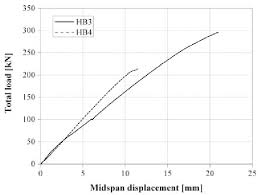# How to Calculate and Solve for Total Load on Composite | Composites

The total load on composite is illustrated by the image below.To compute for total load on composite, two essential parameters are needed and this parameters are Load on Matrix (Fm) and Load on Fibre (Ff).

The formula for calculating total load on composite:

Fc = Fm + Ff

Where:

Fc = Total Load on Composite

Let’s solve an example;
Find the total load on composite when the load on matrix is 20 and the load on fibre is 10.

This implies that;

Fm = Load on Matrix = 20
Ff = Load on Fibre = 10

Fc = Fm + Ff
Fc = (20) + (10)
Fc = 30

Therefore, the total load on composite is 30.

Calculating the Load on Matrix when the Total Load on Composite and the Load on Fibre is Given.

Fm = Fc – Ff

Where:

Fc = Total Load on Composite

Let’s solve an example;
Find the load on matrix when the total load on composite is 34 and the load on fibre is 12.

This implies that;

Fc = Total Load on Composite = 34
Ff = Load on Fibre = 12

Fm = Fc – Ff
Fm = 34 – 12
Fm = 22

Therefore, the load on matrix is 22

Calculating the Load on Fibre when the Total Load on Composite and the Load on Matrix is Given.

Ff = Fc – Fm

Where:

Fc = Total Load on Composite

Let’s solve an example;
Given that total load on composite is 40 and the load on matrix is 25. Find the load on fibre?

This implies that;

Fc = Total Load on Composite = 40
Fm = Load on Matrix = 25

Ff = Fc – Fm
Ff = 40 – 25
Ff = 15

Therefore, the load on fibre is 15.

Nickzom Calculator – The Calculator Encyclopedia is capable of calculating the total load on composite.

To get the answer and workings of the total load on composite using the Nickzom Calculator – The Calculator Encyclopedia. First, you need to obtain the app.

You can get this app via any of these means:

You can also try the demo version via https://www.nickzom.org/calculator

Apple (Paid) – https://itunes.apple.com/us/app/nickzom-calculator/id1331162702?mt=8
Once, you have obtained the calculator encyclopedia app, proceed to the Calculator Map, then click on Materials and Metallurgical under Engineering.Now, Click on Composites under Materials and MetallurgicalNow, Click on Total Load on Composite under CompositesThe screenshot below displays the page or activity to enter your values, to get the answer for the total load on composite according to the respective parameter which is the Load on Matrix (Fm) and Load on Fibre (Ff).Now, enter the values appropriately and accordingly for the parameters as required by the Load on Matrix (Fm) is 20 and Load on Fibre (Ffis 10.Finally, Click on CalculateAs you can see from the screenshot above, Nickzom Calculator– The Calculator Encyclopedia solves for the total load on composite and presents the formula, workings and steps too.